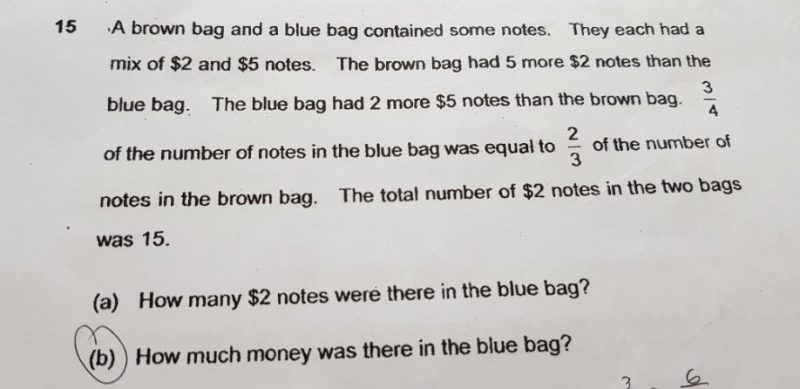# QuestionHello, i need help for this question pls. Thank you

a) Number of \$2 notes = 15
Brown = Blue + 5
Blue + Blues + 5 = 15
Blue = 10/2 = 5 ##

b)
Let 3/4 of blue and 2/3 of brown be 6u
Blue = 8u =>4/4
Brown = 9u =>3/3

Brown has 5 more \$2 notes but 2 less of \$5 notes,
Net difference of notes = 5-2 =3
9u – 8u = 3
u = 3

Blue = 8u = 24 notes,
number of \$2 notes = 5
Number of \$5 notes = 24 – 5 = 19
5X \$2 = \$10
19 X \$5 = \$95
Total = \$100 ###

0 Replies 0 Likes